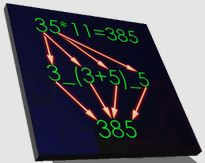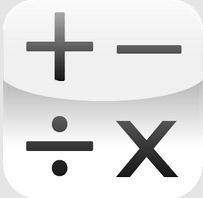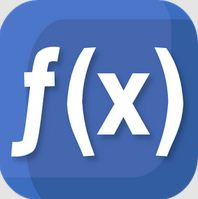# Best Mathematics apps for Android Smartphones

Math as a subject is the most important part of our School, Colleges and University & also in life. If you have any problem related to math calculations and other problem then doesn’t worry here you can get top Best Mathematics apps for Android Smartphone. Using these android math apps you can easily learn Mathematics Shortcuts tricks, Mental Math, Algebra, Vedic MathsGeometric, Logical function, Logarithms, and other important math learning. Below you can get top Best Mathematics apps for Android Smartphones.

In the market several best math apps available for learning math but here you can download most used and best customer reviews and rating android math apps for smartphones.

Best Mathematics Books For All Competitive Exams

Top 10 GCSE Maths apps for iPhone

Best Maths Short cut tricks apps

## Top 10 Best Mathematics apps for Android Smartphones / Android Math apps / Best Math apps1) Math Tricks:

Math Tricks App is the best math app for Addition, Subtraction, Division, Multiplication, etc. This Android math app is also useful for various multiplications & a square number of short cut tricks. Math Tricks app is #1 best Customer review in all learning math app & best maths app for Android.2) Math Workout:

Math Workout app is one of the best math apps for Mental math & Brain Cruncher. You can easily learn Maths calculation such as Addition, Subtraction, Division, Multiplication, etc. This best math app also useful for Adults & kids to learning math so this app is the best maths app for Android.3) Mathematics:

Mathematics app is the best android app for learning math. You can easily learn linear Algebra, Matrices, Vectors, Maxima & Minima, Binomial, Average, Linear equation, Plot function, polynomial, Tangent and important math functions. This android math app is also useful for the Number system and unit conversion. Mathematics app is Best Mathematics apps for Android Smartphones.4) King of Math:

King of math is the best math app for powers & roots, Fractions, Smallest & Largest, Average & Median so this app is one of the best maths apps for Android. using this Android math app you can also learn Addition, Subtraction, Division, Multiplication easily in a few seconds.5) Formulas Mathematics:

Formulas Mathematics app is best math app for Algebra, Geometry, Trigonometry, Analysis, Logic, Analytical Geometry and other math preparation tricks. Using this Android math app 2020, you can easily and fastly solve algebra and Trigonometry questions.6) Math Challenge:

Math Challenge app is one type of math game app for adults and kids. Using this Android math app you can improve you’re your math skills and easily prepare for math. In this math app for Android, you can also test your IQ & Brain.7) Math Expert:

Math Expert app is the best math app for practice Logarithms, Square, Parabola, Cube, and other mathematics functions. Using this Android math app you can easily preparation of Numerical Ability and Complex Numbers. Math Expert app is the Best Mathematics apps for Android Smartphones.8) MalMath: Step by Step Solver:

MalMath app is the best Android math app for the preparation of Integrals, Derivatives, Limits, Equations, Logarithms, Trigonometry and other math android functions. This mathematics app 2020 is also useful for Graph Analysis9) Math Helper Lite: Algebra

Math Helper Lite app is the best math app for the preparation of Vectors, Geometry, Integrals, Matrices, Limits, and Probability, etc. This Android math app contains 10 topics & 60+ Subsection. This Mathematics app also contains an Amazing Scientific Calculator.